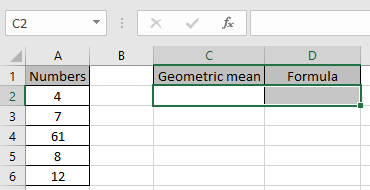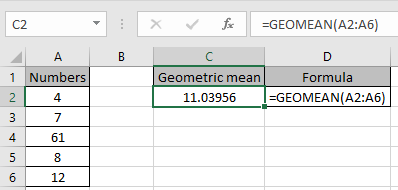# How to use the Excel GEOMEAN function

Geometric mean of n numbers(a,b,c,d,...) is represented by
GM = na*b*c*d*..
GM = (a*b*c*d...)1/2
The GEOMEAN function is a mathematical function to calculate the Geometric mean of the numbers. This function return #NUM! Error if any negative number is supplied.
Syntax:

=GEOMEAN (number1, [number2], ...)

Note: The function ignores text, empty cells and logical_values. This function returns geometric mean.
Let’s understand this function using an example.Use the formula to get geometric mean of the values.

=GEOMEAN(A2:A6)As you can see, the function returns the geometric mean of the values.

Hope you understood how to use GEOMEAN function. Explore more articles on Excel function here. Please feel free to state your query or feedback for the above article.

Related Articles:

How to Calculate Mean in Excel

Excel MEDIAN function

Excel MODE function

Popular Articles:

How to use the VLOOKUP Function in Excel

How to use the COUNTIF function in Excel 2016

How to Use SUMIF Function in Excel

Terms and Conditions of use

The applications/code on this site are distributed as is and without warranties or liability. In no event shall the owner of the copyrights, or the authors of the applications/code be liable for any loss of profit, any problems or any damage resulting from the use or evaluation of the applications/code.